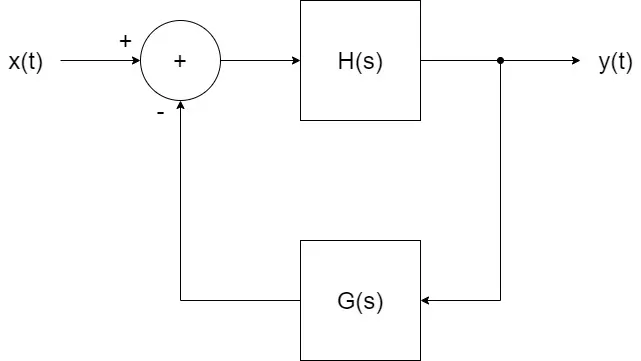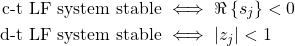# Mathematics - Signals and Systems - Linear Feedback Systems[Image1]

## Introduction

Hey it's a me again @drifter1!

Today we continue with my mathematics series about Signals and Systems to get into Linear Feedback Systems.

So, without further ado, let's dive straight into it!

## Introduction to Feedback

The concepts that we've covered throughout this series are sufficient for the basic analysis of linear feedback systems. But, let's first try to understand what Feedback is and why it's so useful.

Feedback is something that sometimes even comes naturally, like the audio speaker feeding back to the microphone. Too strong of a feedback leads to system instability (distortion in the case of mics). But, just the right amount can be useful for controlling or enhancing the system performance. Feedback applications include:

• Amplifier design : compensate for uncertainties in element characteristics such as the frequency response.
• Stabilizing unstable systems
• in a control system some disturbances and parameters might not be specified accurately.
• use error signal to find and minimize between the desired and actual value.
• when using feedback the system is less-sensitive then an open-loop control system

In modern control systems, digital, and thus discrete-time, feedback systems are used.

## Linear Feedback System Analysis

In the case of continuous-time LF systems, the main analysis tool is the Laplace transform, whilst in the case of discrete-time LF system it's the Z-Transform. Either way, in both cases the so called basic feedback equation describes the overall system function of a feedback system in terms of two paths:

• forward path (system response)
• feedback path (system feedback)

Suppose H(s) is the forward path equation and G(s) the feedback path equation, then a basic feedback system can be visualized graphically as follows:Or mathematically, the system feedback equation of this continuous-time LF system is:In the case of discrete-time, s simply changes into z.

There are different conditions for stability in C-T and D-T:

• a continuous-time system must have all of its poles in the left-half s-plane
• a discrete-tiem system must have all of its poles inside of the unit circle

Mathematically, these conditions can be written as:From these conditions it's also possible to come to the conclusion that the combined gain of the forward and feedback paths must be less than unity (1). Thus, if K1 and K2 are the forward and feedback path gains respectively, then:## RESOURCES:

### Images

1.Block diagrams and other visualizations were made using draw.io

## Previous articles of the series

### Laplace and Z Transforms

• Laplace Transform → Laplace Transform, Region of Convergence (ROC)
• Laplace Transform Properties → Linearity, Time- and Frequency-Shifting, Time-Scaling, Complex Conjugation, Multiplication and Convolution, Differentation in Time- and Frequency-Domain, Integration in Time-Domain, Initial and Final Value Theorems
• LTI System Analysis using Laplace Transform → System Properties (Causality, Stability) and ROC, LCCDE Representation and Laplace Transform, First-Order and Second-Order System Analysis
• Exercises on the Laplace Transform → Laplace Transform and ROC Examples, LTI System Analysis Example
• Z Transform → Z Transform, Region of Convergence (ROC), Inverse Z Transform
• Z Transform Properties → Linearity, Time-Shifting, Time-Scaling, Time-Reversal, z-Domain Scaling, Conjugation, Convolution, Differentation in the z-Domain, Initial and Final value Theorems
• LTI System Analysis using Z Transform → System Properties (Causality, Stability), LCCDE Representation and Z Transform
• Exercises on the Z Transform → Z Transform and ROC Examples, ROC from Conditions, LTI System Analysis Example
• Continuous-Time to Discrete-Time Design Mapping → Discrete-Time System Design Techniques (Mapping from Derivatives to Differences, Mapping using Impulse Invariance), First- and Second-Order Systems and Z-Transform
• Butterworth Filters → Butterworth Filter Parameters, Equation and Pole-Zero Plot, Mapping using Impulse-Invariance and Bilinear Transformation

## Final words | Next up

And this is actually it for today's post!

Next up will be System Feedback Exercises...

See Ya!Keep on drifting!

H2
H3
H4
3 columns
2 columns
1 column